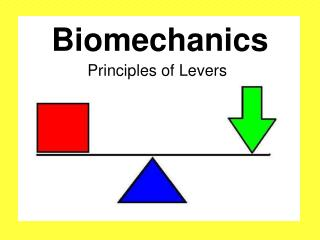# Biomechanics - PowerPoint PPT PresentationDownload PresentationBiomechanics

BiomechanicsDownload Presentation## Biomechanics

- - - - - - - - - - - - - - - - - - - - - - - - - - - E N D - - - - - - - - - - - - - - - - - - - - - - - - - - -
##### Presentation Transcript

1. Biomechanics Principles of Levers

2. Principles of Levers Aim: To understand how the three types of levers work and appreciate the advantages/disadvantages of each one.

3. Principles of Levers Key Vocabulary • Lever • Fulcrum • Resistance • Effort

4. Levers are… • Simple machines that help us apply force. • Rigid structures, hinged at some part with forces applied at two other points.

5. We have levers within our body, and also use them externally for many different reasons.

6. All levers have three parts: • Fulcrum • Resistance • Effort

7. Fulcrum The pivot point.

8. Resistance(or load) The weight that needs to be moved.

9. Effort The force that is applied to move the resistance (or load).

10. Force Arm & Resistance Arm • With levers, there are also a FA and a RA. • Force Arm (FA) = The distance from where a force is applied to the fulcrum. • Resistance Arm (RA) = The distance from where a resistance acts to the fulcrum. • The relationship between force arm length, with respect to the resistance arm length is important when we consider what the function of the lever is. That is, are we after maximum speed with a given effort or moving a greater resistance with a given force?

11. Functions of a Lever Levers perform two main functions: To increase the resistance (or force) that can be moved with a given effort e.g. a crowbar. To increase the velocity at which an object will move with a given force. E.g. A golf club.

12. Classes of Levers There are 3 classes of levers. Each is structured in a different manner and has different advantages.

13. First Class Levers The fulcrum lies between the effort and the resistance.

14. First Class Levers Using a crowbar to move a rock.

15. First Class Levers Using a hammer to pull out a nail.

16. First Class Levers A see-saw.

17. First Class Levers Up and down movement of the head about the atlas joint.

18. First Class Levers Pulling an oar in a row boat.

19. Second Class Levers The resistance lies between the fulcrum and the point of effort.

20. Second Class Levers The movement of the foot when walking. (the calf muscle provides the effort and the ball of the foot is the pivot)

21. Second Class Levers Opening a door by the handle.

22. Second Class Levers Opening a bottle with a bottle opener.

23. Second Class Levers Pushing a wheel barrow.

24. Third Class Levers The effort lies between the resistance and the fulcrum.

25. Third Class Levers Biceps curl.

26. Third Class Levers Fishing with a rod.

27. Third Class Levers Swinging a bat to hit a ball.

28. Application Long levers provide amplified power at the expense of speed of movement of the object being moved. Examples: • Using a jack to lift a crashed car. • Using a knife to get the lid off a tin. • Using a driver in golf.

29. Application Short levers provide speed at the expense of power Example: Levers designed to accelerate objects include: - Most body limbs used for throwing or kicking because the muscle inserts near the pivot point. - Using a 9-iron in golf.

30. Application • Can you think of sporting examples where there are longer and shorter levers? • What happens to the effectiveness of a lever if the fulcrum is moved closer or further away from the load?

31. Revision Question One: Fill in the blanks Levers are simple machines which helps us apply ____(a)____ . They have ___(b)___ parts. The ___(c)___ is the pivot point. The ___(d)___ (or load) is the weight that needs to be moved. The ___(e)___ is the force that is applied to move the resistance.

32. Revision Question Two: Label the diagram

33. Revision Question Three:First, Second or Third Class? (a) (b) (c) The effort lies between the resistance and the fulcrum. The fulcrum lies between the effort and the resistance. The resistance lies between the fulcrum and the point of effort.

34. Revision Question Three:First, Second or Third Class? (d) (e) (f)

35. Revision Question Three:First, Second or Third Class? (g) (h) (i) (j)

36. Revision Question Three:First, Second or Third Class? (k) (l) (m) (n)

37. Application • How would you change your grip on a softball bat if you wanted to hit the ball with more force? Experiment with this with a partner. • With a partner find another physical activity example that will be able to demonstrate application of the principles of levers, showing what happens when you change the length of your lever.